# Spherical harmonics

(diff) ← Older revision | Latest revision (diff) | Newer revision → (diff)

of degreeA restriction of a homogeneous harmonic polynomial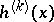of degreeinvariablesto the unit sphere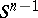of the Euclidean space,. In particular, when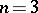, the spherical harmonics are the classical spherical functions.

Let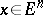,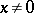,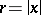,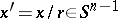. The basic property of spherical harmonics is the property of orthogonality: If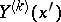and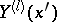are spherical harmonics of degreeand, respectively, with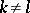, then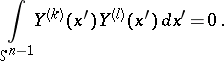The simplest spherical harmonics are the zonal spherical harmonics. For any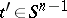and any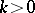, a zonal spherical harmonic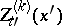exists which is constant on any parallel of the spherethat is orthogonal to the vector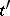. The zonal spherical harmonicsdiffer from the Legendre polynomials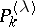, when, or from the ultraspherical polynomials, when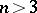, only by a constant factor: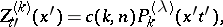where the polynomialsare defined, when, by the generating function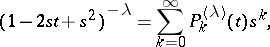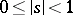,,. The polynomials,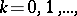are orthogonal with weightand form an orthogonal basis of the space. Ifis a function in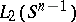with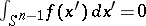, then there is a unique set of spherical harmonics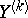such that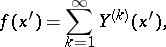where the series converges in the norm of.

Expansions in spherical harmonics are largely analogous to expansions in Fourier series, of which they are essentially a generalization. The homogeneous harmonic polynomialsare sometimes called spatial spherical harmonics. By virtue of the homogeneity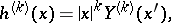spherical harmonics are sometimes also called surface spherical harmonics.

How to Cite This Entry:
Spherical harmonics. Encyclopedia of Mathematics. URL: http://encyclopediaofmath.org/index.php?title=Spherical_harmonics&oldid=12872
This article was adapted from an original article by E.D. Solomentsev (originator), which appeared in Encyclopedia of Mathematics - ISBN 1402006098. See original article